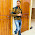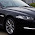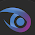# Merge Sort Using C++

Merge sort is a sorting algorithm for sorting elements of array in either ascending or descending order. Conceptually, a merge sort works as follows:

a) Divide the unsorted list into n sub lists, each containing 1 element(a list of 1 element is considered sorted).

b) Repeatedly merge sub lists to produce new sorted sub lists until there is only 1 sub list remaining. This will be the sorted list.
```
```
`/* C++ Program to implement Merge Sort */ `
` `
```#include<iostream>
using namespace std;

void merge_sort(int [],int ,int );
void merge(int [],int,int ,int );

int main()
{
int n;
cout<<"Enter the size of the array"<<endl;
cin>>n;
int a[n];
cout<<"Enter the elements in the array"<<endl;
for(int i=1;i<=n;i++)
{
cin>>a[i];
}

cout<<"sorting using merge sort"<<endl;
int p=1,r=n;

merge_sort(a,p,r);

cout<<"sorted form"<<endl;
for(int i=1;i<=n;i++)
{
cout<<"a["<<i<<"]="<<a[i]<<endl;
}
return 0;
}

void merge_sort(int a[],int p,int r)
{
int q;
if(p<r)
{
q=(p+r)/2;
merge_sort(a,p,q);
merge_sort(a,q+1,r);
merge(a,p,q,r);
}
}

void merge(int a[],int p,int q,int r)
{
cout<<"Entered merge"<<endl;
int n1=q-p+1;
int n2=r-q;
int L[n1+1];
int R[n2+1];
for(int i=1;i<=n1;i++)
{
L[i]=a[p+i-1];
}
for(int j=1;j<=n2;j++)
{
R[j]=a[q+j];
}
L[n1+1]=999;
R[n2+1]=999;
int i=1, j=1;
for(int k=p;k<=r;k++)
{
if(L[i]<=R[j])
{
a[k]=L[i];
i=i+1;
}
else
{
a[k]=R[j];
j=j+1;
}
}
}```
` `
`//Output of the above program`
` `Merge Sort Using C++
```

```
Related Programs:-

Heap Sort using C++

#### 10 comments:

1.This code has a number of problems, the worst are:

- It uses 999 as a end marker so if the array contains larger values than this it might be incorrectly sorted or the program might even crash. There shouldn't be any end marker used in merge sort.

- It allocates temporary arrays on the stack so it can only short arrays. It should allocate the temporary array (which is required for merge sort) on the heap.

- It's not generic so it only works on arrays of ints. It should be able to sort any standard collection with random access iterators containing any element type supporting the less than operator.

- It's inefficient and performs unnecessary copying and comparisons. A minimum number of copies and comparisons should be performed.

I know the intentions are good, but I would considering this really bad C++ code and if I saw this in production code I would rewrite it from scratch.

1.What about this algo?

http://www.coders-hub.com/2013/04/merge-sort-progam-in-c.html

2.Well it solves the two first problems. It's still a bit inefficient (too many heap allocations and unnecessary copying and comparisons) and since it's C code it's not generic.

3.Also it leaks memory and assumes sizeof(int) == 2.

4.Than share your code Sir and help others.

2.This comment has been removed by a blog administrator.

3.Poorly written. You may upload the below if you like.

#include < iostream >
#include < vector >

void GetData (std::vector &array);
void DisplayData (std::vector array);
void Merge (std::vector &left, std::vector &right, std::vector &array);
void Sort (std::vector &array);

int main()
{
std::cout<<"Enter array (-1 to exit)\n";
std::vector array;
GetData(array);

std::cout<<"Size of array is "< &array)
{
int num = 0;
while (num != -1)
{
std::cin>>num;
if (num != -1)
array.push_back(num);
}
}

void DisplayData(std::vector array)
{
int i = 0;
while (i < array.size())
{
std::cout< &left, std::vector &right, std::vector &array)
{
//remove all elements from the vector
array.clear();

//i for the left array, j for the right
int i = 0, j = 0;

while (i < left.size() && j < right.size())
{
if (left.at(i) <= right.at(j))
array.push_back(left.at(i++));
else
array.push_back(right.at(j++));
}

//if some elememts still left in either left or right vectors
while (i < left.size())
array.push_back(left.at(i++));

while (j < right.size())
array.push_back(right.at(j++));
}

void Sort(std::vector &array)
{
//base condition
if(array.size() < 2)
return;

//store elements in the left and right sub lists
std::vector left(array.begin(), array.begin() + array.size() / 2);
std::vector right(array.begin() + array.size() / 2, array.end());

//recursive functions
Sort(left);
Sort(right);
Merge(left, right, array);
}

4.This is exactly the same algorithm as the pseudocode from the Cormen book I have. The only different is for the sentinel value, we use infinity instead of 999. I'm getting a seg fault though and I see why. It's if one subarray hits the end before the other. I added extra conditions in the last for loop and i'm still hitting the seg fault

5.Thanks Bro. Very Nice work done

6.merge sorting example codes on c programming
Merge sort